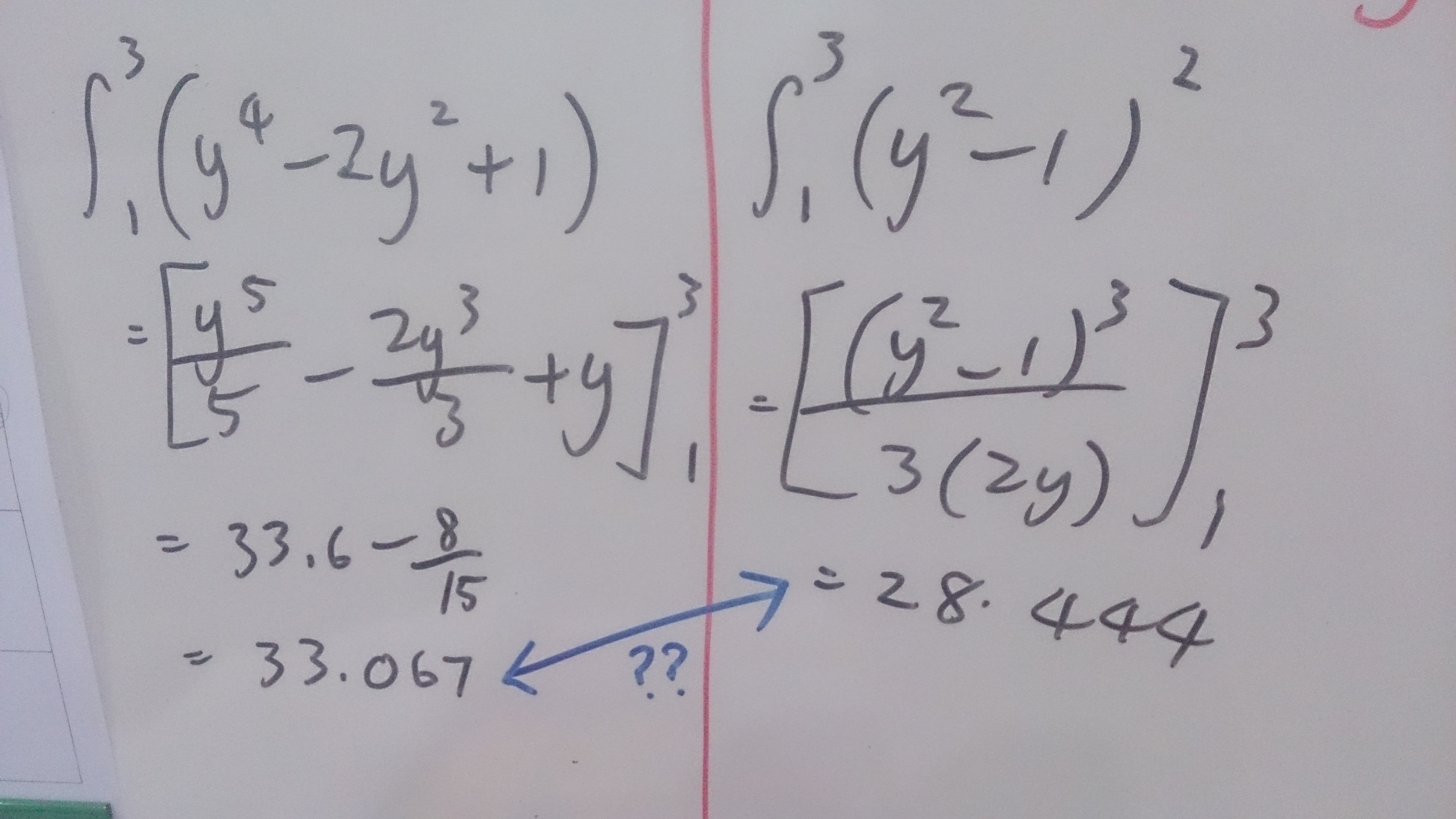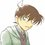# Extremely Weird Integration.(y^2 - 1)^2 = y^4 - 2y^2 + 1 . Aren't both equation the same? Why after integration , the answers become different?

Can anyone explain it?Note by 柯 南
5 years, 11 months ago

This discussion board is a place to discuss our Daily Challenges and the math and science related to those challenges. Explanations are more than just a solution — they should explain the steps and thinking strategies that you used to obtain the solution. Comments should further the discussion of math and science.

When posting on Brilliant:

• Use the emojis to react to an explanation, whether you're congratulating a job well done , or just really confused .
• Ask specific questions about the challenge or the steps in somebody's explanation. Well-posed questions can add a lot to the discussion, but posting "I don't understand!" doesn't help anyone.
• Try to contribute something new to the discussion, whether it is an extension, generalization or other idea related to the challenge.

MarkdownAppears as
*italics* or _italics_ italics
**bold** or __bold__ bold
- bulleted- list
• bulleted
• list
1. numbered2. list
1. numbered
2. list
Note: you must add a full line of space before and after lists for them to show up correctly
paragraph 1paragraph 2

paragraph 1

paragraph 2

[example link](https://brilliant.org)example link
> This is a quote
This is a quote
    # I indented these lines
# 4 spaces, and now they show
# up as a code block.

print "hello world"
# I indented these lines
# 4 spaces, and now they show
# up as a code block.

print "hello world"
MathAppears as
Remember to wrap math in $$ ... $$ or $ ... $ to ensure proper formatting.
2 \times 3 $2 \times 3$
2^{34} $2^{34}$
a_{i-1} $a_{i-1}$
\frac{2}{3} $\frac{2}{3}$
\sqrt{2} $\sqrt{2}$
\sum_{i=1}^3 $\sum_{i=1}^3$
\sin \theta $\sin \theta$
\boxed{123} $\boxed{123}$

Sort by:

The integration of $(y^2-1)^2$ is incorrect. You seem to have used something similar to the chain rule of differentiation, which is not applicable to integration. The correct way to do it would be to expand and continue as you did on the left hand side.

- 5 years, 11 months ago

(I'm a student in malaysia) but the formula is in d text book ? Are there ways to do that without expanding ?

- 5 years, 11 months ago

Could you please post a picture of the formula in your textbook? And no, there's no quicker way. However, if you write the integral as the limit of an infinite summation, you will obtain the correct answer but after a lot of tedious calculation.

- 5 years, 11 months ago

Sorry , not text book , it's reference book. Maybe they wrote it wrong.

Thank you so much :D , i wondered about it for so many days

- 5 years, 11 months ago

- 5 years, 11 months ago

You have performed the integral of $(y^2-1)^2$ incorrectly.

- 5 years, 11 months ago

What is the correct steps?

- 5 years, 11 months ago

I check both so many times , can't find anything wrong with it.

- 5 years, 11 months ago

You can not divide by 2y, that only works when the derivative of the inside is a constant. In your case it was 2y which is not a constant. Hope that helps

- 5 years, 11 months ago

Thank you. That was a clear explanation !

(btw , why 2y is not constant ? Isit because y^2 can be ± ？

- 5 years, 11 months ago

The integration of (y^2 - 1)^2 is incorrect. therefore you got different computation

- 5 years, 11 months ago